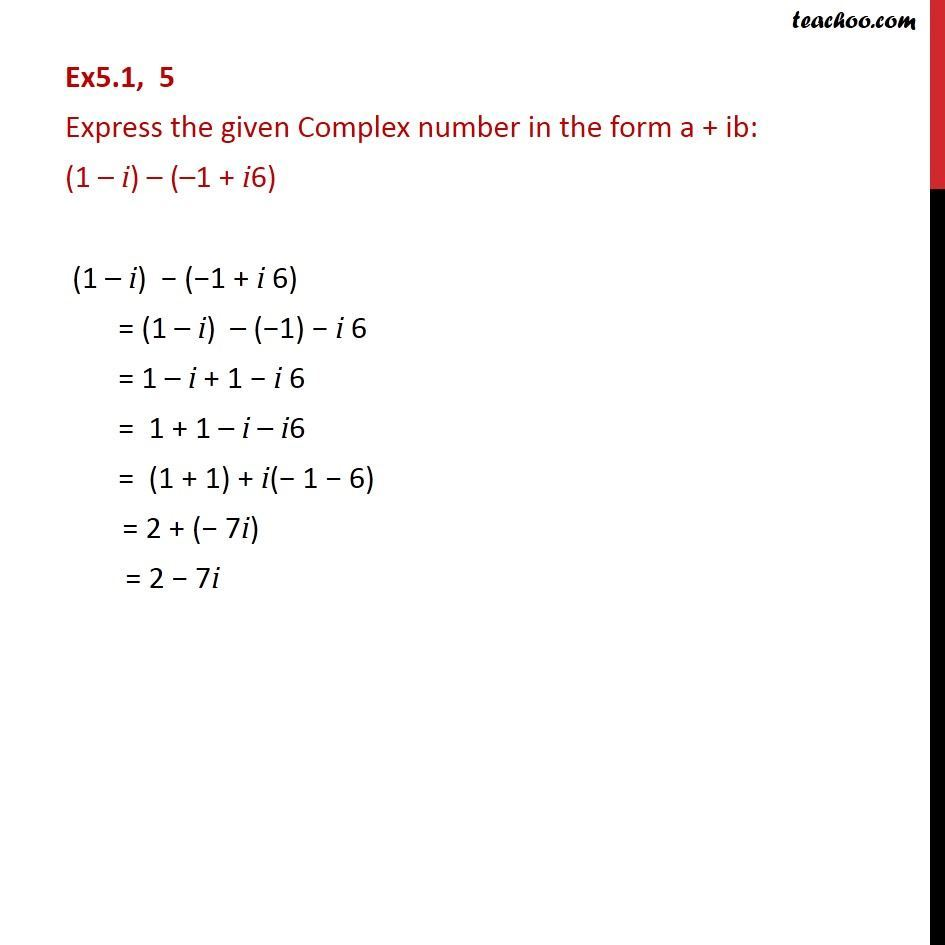Convert to a + ib form

Chapter 4 Class 11 Complex Numbers
Concept wiseLearn in your speed, with individual attention - Teachoo Maths 1-on-1 Class

### Transcript

Ex5.1, 5 Express the given Complex number in the form a + ib: (1 – i) – (–1 + i6) (1 – i) − (−1 + i 6) = (1 – i) – (−1) − i 6 = 1 – i + 1 − i 6 = 1 + 1 – i – i6 = (1 + 1) + i(− 1 − 6) = 2 + (− 7i) = 2 − 7i